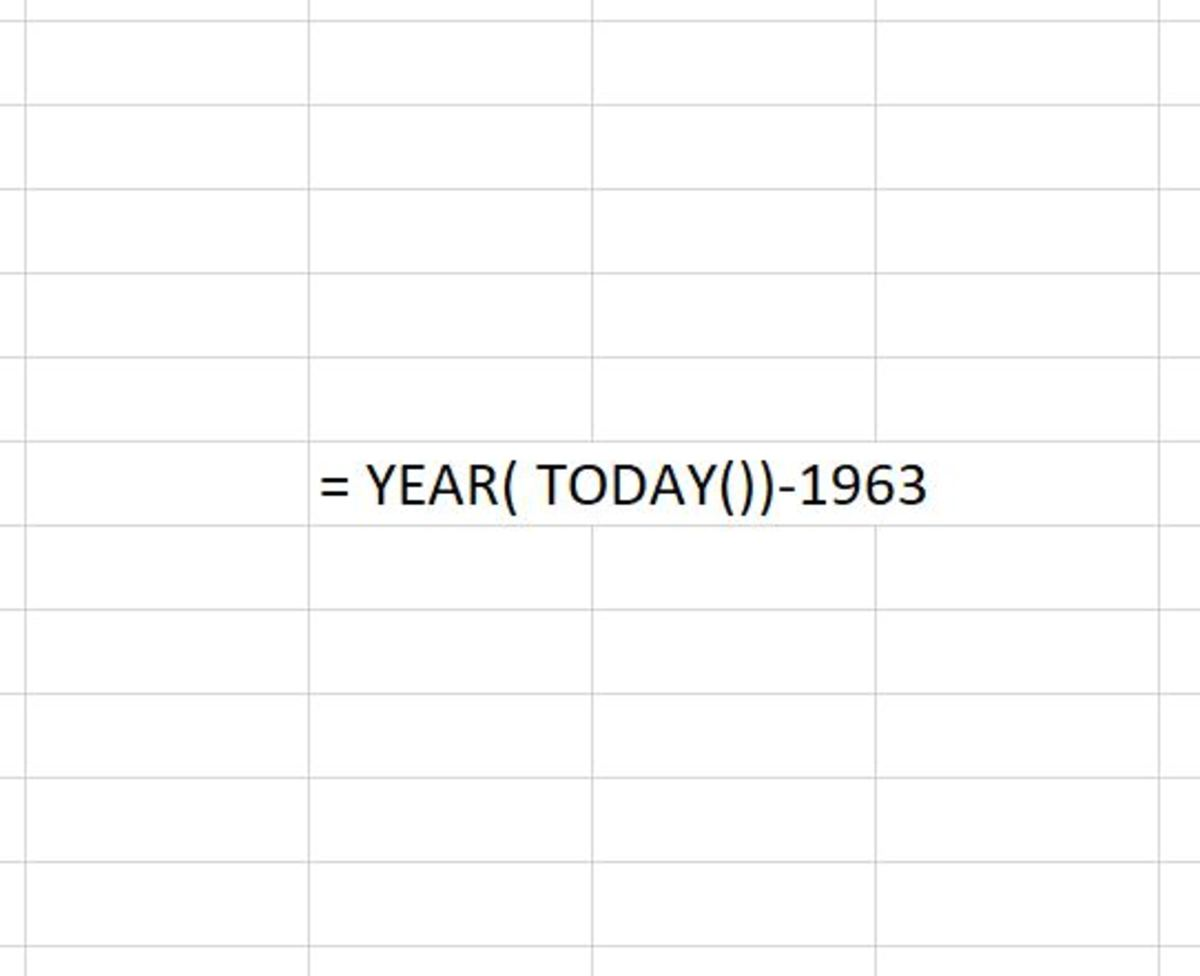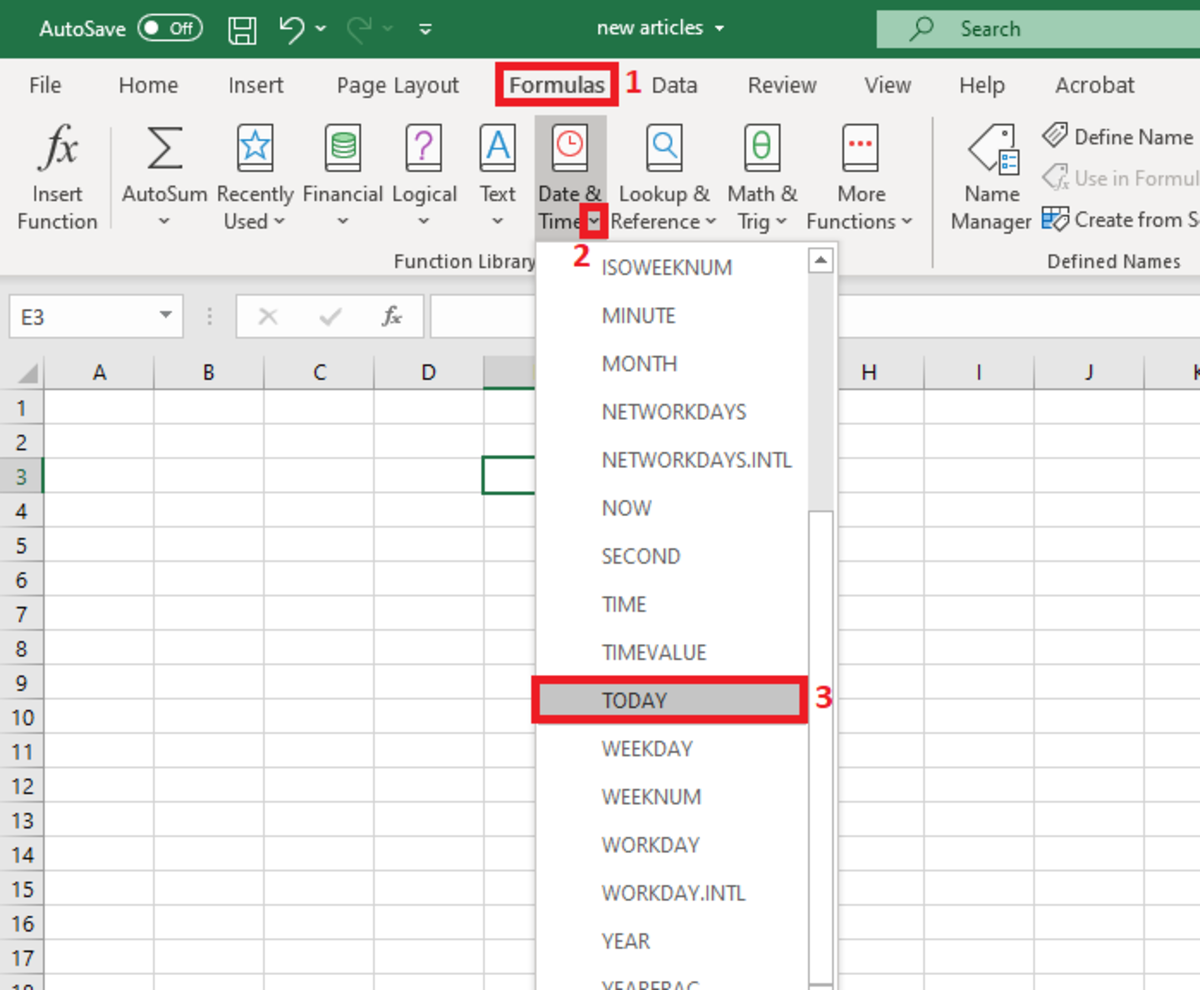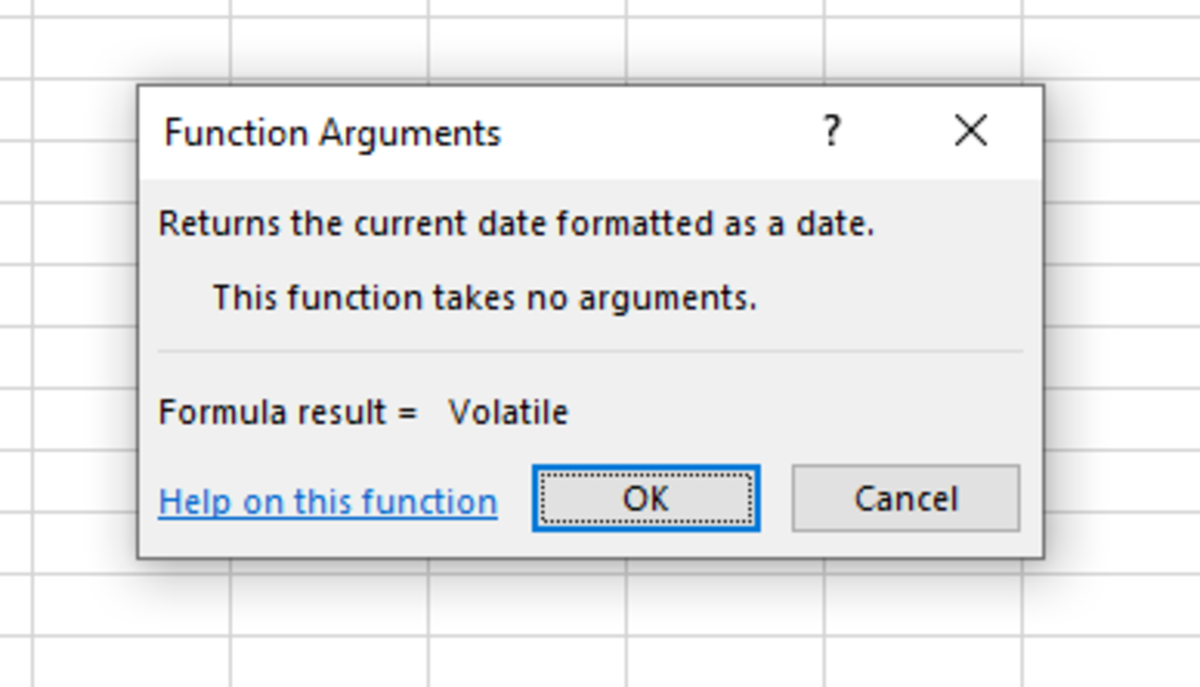# How to Use the TODAY Function in Excel

Joshua earned an MBA from USF and he writes mostly about software and technology.The TODAY function is used in tandem with other functions to determine past and future dates as well as determine the current date in its simplest form. Created by Joshua Crowder

## The Purpose of the TODAY Function

The TODAY function is used to return the current date as long as the cell it is used in is formatted as a date. This function is very useful when you have other formulas that depend on the current date to make accurate calculations. Such a calculation may involve using today's date to figure out the remaining days until something is due. It could involve something a little more complex in an Excel workbook like when to trigger a macro process.

Nevertheless, the TODAY function is very useful, and it's worth learning the various applications that are supported by this function.

## The Syntax

The TODAY function needs to be inputted like a formula to work properly. To enter it, start by clicking into a cell and typing "=TODAY()" into the cell. After the closed parenthesis click enter. Unlike many other functions, this one requires no arguments. The formula simply returns the current date as long as the cell that it is in has date formatting assigned to it.

## Inserting the Function

The TODAY function can be inserted into a spreadsheet with the use of an insert tool. I don’t recommend using this method due to the simplicity of the syntax. The insert option could be used in the event that one forgets how to use the function syntax. The insert tool will basically just write the function for you.

To use this method a cell must first be selected. Next, the formulas tab is selected and the "Date & Time" button on the Excel ribbon needs to be selected. Choose the TODAY function from the drop-down list.Selecting the TODAY Function From the Formulas TabCreated by Joshua Crowder

After the message box appears telling you that the function requires no arguments, click OK and the current date should appear. If not, then select the cell and go back to the home tab. In the numbers section, you should be able to change the formatting to date.When this box appears after inserting a function it is merely to make sure you understand that the function does not require any data. Created by Joshua Crowder

## TODAY Function Examples

The following examples are just a few ways that the today function can be used in a worksheet.

=TODAY()

• Displays that current date. For this example and the following examples, the format of the date will be determined by the format that is set for the cell that this function is used.

=TODAY()-7

• Displays the date that was one week in the past. You can also go any number of days into the past as you wish.

=TODAY()+7

Scroll to Continue
• Displays the date that will come one week into the future. You can also go any number of days into the future as you wish.

= YEAR(TODAY())-1963

• Displays the number of years past by subtracting a certain year from today's date. For this example, it would be more practical to use a cell reference for the year if this example were used in a spreadsheet application.

=DAY(TODAY())

• Determines the day in a number format of the current month. So, if it was July 5th, this formula would display the number 5 in a cell.

## TODAY Function Practical Application Example

For example, let's take a look at a situation where one may use the TODAY function on a day-to-day basis. Take into consideration the table in the illustration below. The information is part of a spreadsheet that is dedicated to tracking laboratory specimens related to plastics laboratory testing.

## Spreadsheet Column Descriptions

The table below shows descriptions of the columns of the spreadsheet example. Three dates are tracked for which two will be used to find out to determine a binomial answer that results from an if statement and how many days have passed till a certain date.

ColumnDescription

Date the raw plastic material arrived.

Test Needed by

Date that test specimen were molded and when the curing process starts.

Date Molded

Date that the test plaques were molded.

2 Day Cure?

Formula to determine.

Days Till Due Date

Formula to determine.

To determine if a two-day cure has occurred, the today function uses the current date and subtracts the mold date. This type of calculation will give the difference between the two dates resulting in the number of days that have passed.

Since if the formula is looking for a number greater than 2, a "Yes" appears in the cell. Otherwise, a "No" would appear until the following days trigger the TODAY function to result in a difference greater than two.

The calculation that determines the days till the due date is straightforward. The cell reference C4 represents the due which is subtracted by today's date. The result will be the amount of days left until the due date occurs.